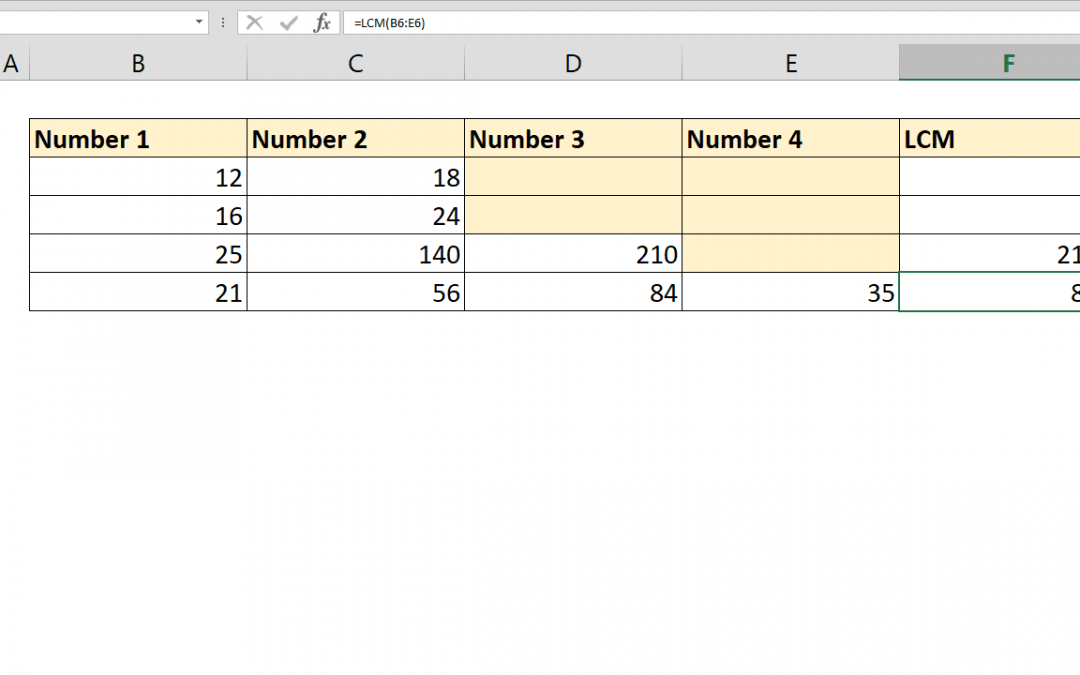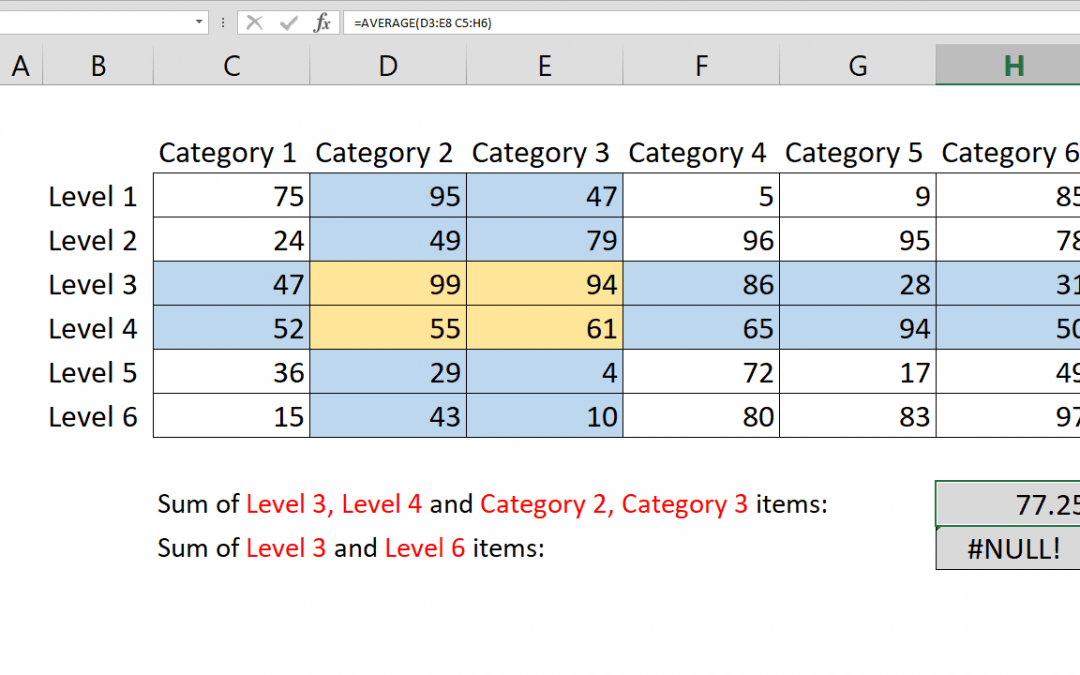## How to count characters in Excel in a range

The LEN function is used to count characters in Excel. But it doesn’t support ranges or arrays to easily count total number of characters in a range. This article shows 2 ways to count characters in Excel in a range which uses SUMPRODUCT and SUM along with LEN....## How to calculate Least Common Multiple

Excel has a built in function to calculate Least Common Multiple (LCM) of two or more integers. The function is also called LCM. Syntax =LCM(number1, [number2], …) Steps Start with =LCM( Type or select cells or range that includes integers B6:E6 Type ) to close the...## How to calculate Greatest Common Divisor (GCD)

To calculate Greatest Common Divisor (GCD) in Excel is very straightforward. Excel has a built in function to calculate the greatest common divisor of two or more integers. The function called GCD. Syntax =GCD(number1, [number2], …) Steps Start with =GCD( Type or...

## Windows Character Set (1252)

Windows Character Set is a one byte character encoding system derived from Latin alphabet. The character set shares same characters up to first 128 character with ASCII character encoding. Excel’s the CHAR function uses this characters set for Windows versions....

## MAC OS Character Set

Mac OS Character Set is based on one byte character encoding system, Mac-Roman. The character set shares same characters up to first 128 character with ASCII character encoding. Excel’s the CHAR function uses this characters set for Mac versions. Code...## How to use double quotes in Excel formulas

Excel uses double quote character to identify text, you need to a different approach. This article shows you How to use double quotes in Excel formulas in two ways: with and without a function. Syntax =”””” =CHAR(34) How There are 2 ways to use...## How to calculate AVERAGE with intersecting tables

This article shows how to calculate average with intersecting tables using AVERAGE function and intersect operator, the space character (” “). Syntax =AVERAGE(first range second range) Steps Type =AVERAGE( function Select or type the first range reference...## Count values with Excel intersect operator

Excel intersect operation is handled by the space character (” “). You can intersect multiple rages by space in range supporting formulas. This article shows how to COUNT intersecting ranges with COUNT function and Excel intersect operator. Syntax...## How to calculate SUM with intersecting tables

This article shows how to calculate sum with intersecting tables using SUM function and intersect operator, the space character (” “). Syntax =SUM(first range second range) Steps Type =SUM( function Select or type the first range reference that includes...